Search

# Fundamentals of Bayesian Meta-Learning Algorithms

Written by Jennifer Yuan

In this Meta-Learning article, we will discuss Bayesian Meta-Learning. In specific, we will discuss ways to apply Bayesian inference to Meta-Learning. The answers are simply true-or-false that hardly captures the uncertainty in the true world. Integrating Bayesian inference will bridge the gap.

## Bayesian Meta-Learning

Many methods mentioned are point estimation — we find an optimum that minimizes the loss. In the Bayesian world, we answer a slightly different question — what are the probabilities of different possibilities. So it answers the probability distribution p(y) instead of what y is. The Bayesian method reasons about uncertainty and model the real world better. For example, we may say a person is late with an 80% chance that the traffic is bad and a 20% chance that he/she overslept. Absolutely “right” is just plain wrong most of the time. It throws away numerous important information.

Unfortunately, Bayesian inference is often intractable that requires simplifications and approximations. Let’s demonstrate the difficulty in an example quickly. Say, μ and z are random variables with a normal and a multimodal distribution respectively. If xᵢ is sampled from equation 2(b) below what will the posterior p(μ, z | x) be? Both μ and z are simple distributions, so we may expect this can be solved with Bayes’ Theorem easily. Nevertheless, as shown below, the integral is complex and makes the posterior intractable. In fact, intractable is the norm in many of Bayes’ calculations.Modified from source

Because the posterior is not tractable, we want to approximate it directly. That leads us to variational approximations.

### Variational Inference

Posterior calculations can be nasty. Variational inference is about approximating a posterior (the blue line below), say p(z|evidence=X), with some easy to manipulate distribution q like the Gaussian (red line).Here, let’s discuss variational inference in the context of meta-learning. The graphical models below will be our starting points. The solid arrow defines the dependency. θ →𝜙means task 𝜙depends on meta-learner θ. The dotted lines denote variational approximations. For example, in the right diagram, we approximate p(𝜙ᵢ | X_support, Y_support , θ) with q. (If you have issues with the Graphical model concept, don’t worry too much. It mainly documents the dependency better in our discussion.)Variational inference is a huge topic. So we are not trying to explain it fully. Otherwise, it will take a lot of time. If you need to understand more, this general article on variational inference will be a helpful start later.

The training objective is to maximize the marginal log-likelihood log p(Dᵢ). Let’s marginalize (integrate) it with all possible θ and 𝜙ᵢ.In the equation above, we approximate p(θ), p(𝜙₁), p(𝜙₂), p(𝜙₃)with variational approximators using models q parameterized by ψ, λ₁, λ₂, λ₃ … respectively. For example, these variational approximators can be Gaussians and ψ, λ₁, λ₂, λ₃ … represent their means and variances. The equation above establishes the evidence lower bound. Without proof here, in variational inference, minimizing this lower bound is equivalent to maximizing the marginal log-likelihood. In fact, the lower bound objective is easier because the marginal p(Dᵢ) usually involves nasty integrations. Therefore, the equation below is our training objective.In the first term above, we want the adapted task model 𝜙ᵢ to fit the observed task samples the best. If 𝜙ᵢ is the parameters of a classifier, we want the classifier to make predictions that match the true labels. The second term and the third term penalize the difference between p and the approximator q for p(𝜙ᵢ|θ) and p(θ) respectively.This sounds a little bit odd that we need to know p( 𝜙ᵢ|θ) and p(θ) in the first place. So this equation is just a starting point. In fact, it will be further simplified using more assumptions and other changes may be made for practical purposes.

For example, many Meta-Learning algorithms assume both p( 𝜙ᵢ|θ) and p(θ) are some simple distributions, like Gaussians. The main task is just finding its parameters (the mean and the variance). Let’s start our discussion with the equation below: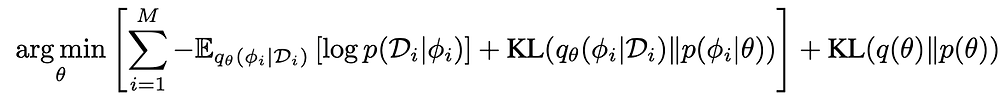where 𝜙ᵢ is the learner for task i and θ is the meta-learner.

In this formularization, we often start with some random or educated guesses for the parameters on p(θ), say mean = 0 and variance = 1 for a Gaussian. Then, we adapt θ to become 𝜙ᵢ using the observed task samples. So, with p(θ) fixed, we approximate p( 𝜙ᵢ|θ, Dᵢ) with a variational posterior q(𝜙ᵢ) in the inner loop. One of the challenges in this formularization is to approximate the posterior underlined in red below.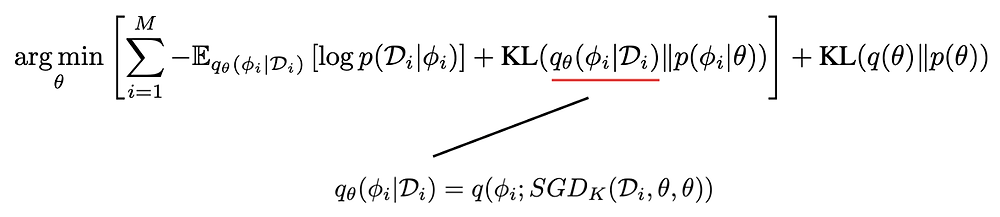One popular approach starts initializing 𝜙ᵢ as θ. Then, we update the model parameters for 𝜙ᵢ using a k-th step gradient descent (SGDk) with a loss function based on the likelihood of predicting the true labels as well as the discrepancy in the posterior estimation.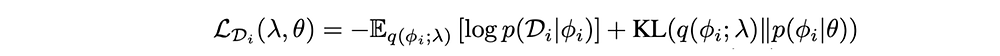Don’t worry about the fine details for now since many variants remain and we will cover them in detail later. In this example, after a K-step gradient descent, SGD returns the mean and the variance of the Gaussian qθ that approximates p(𝜙ᵢ|Dᵢ)Once we estimate q(𝜙ᵢ) for one or more tasks in the inner loop, we fix it and optimize θ. This strategy is very common in ML. The optimization problem is much easier to solve if we have two sets of latent variables and when one is fixed, the other can be optimized easily (or vice versa). But the problem becomes intractable when both are unknown. So, the strategy is optimizing one latent variable with the other fixed in an inner loop while reversing the role in the outer loop. Both will improve gradually which leads to a local optimum. Intuitively, we refine the posterior q(𝜙ᵢ) with observed samples. Then we optimize θ that leads us to these 𝜙ᵢ models better.

### Amortized Variation Inference

There are alternatives to the SGD approach in approximate a posterior. But many variational posterior is too hard to be modeled manually.Amortized Posterior Inference introduces a parameterized model that outputs the variational parameters of the approximate posteriors. For example, we can assume p( 𝜙ᵢ | θ) to be a Gaussian and use a NN (neural net) to predict the mean and variance of this Gaussian distribution. To train this NN, we use gradient descent with the loss function for the variational inference.

The diagram below train a NN to produce a Gaussian distribution over h to act as the context of the meta-training data.Then, 'h' is used in another NN in making predictions. For example, h may contain 1,000 components in which each component represents a parameter in an MLP classifier. Once, h is calculated from the support, each component in h will contain the mean and the variance of a corresponding parameter in the MLP. We sample value from a Gaussian with this mean and variance and use it for that parameter in the MLP.

### Variational Auto-Encoder

Let’s go through a more detailed example in showing how we model variational posterior q(z) with a NN. First, we model the prior and the likelihood in the Bayes’ Theorem. Even latent factor z is learned, it is some abstract concepts we introduce and therefore, we do have a lot of freedom in choosing its distribution. Here, the prior p(z) is chosen to have a multivariate Gaussian with mean 0 and diagonal covariance I. Uniform distribution is another popular choice. And let’s assume the likelihood can be modeled by a multivariate Gaussian with μ and σ².Equation source

As shown above, μ and σ² will be predicted by a NN (neural net). For example, this NN can be in the form of an MLP decoder p(x|z). These model parameters for p(z) and p(x|z) are collectively named as the generative parameters θ. In our example, θ includes (0, I, W₃, W₄, W₅, b₃, b₄, b₅). Next, let’s estimate the variational posterior q with a multivariate Gaussian (parameterized by 𝜙) with mean predicted by an MLP encoder p(z|x).To sample a value for z, we can apply the reparameterization trick below. This formularization is differentiable and therefore, it is gradient descent friendly and becomes handy in many algorithms.Without much justification, the corresponding loss function L(θ, 𝜙; xⁱ ) that maximizes the marginal likelihood of sample i (log p_θ(xⁱ )) is:Equation source

With the reparameterization trick, the loss function is differentiable w.r.t. θ and 𝜙. Therefore, we can apply Gradient Descent to optimize them. Below is the skeleton of the algorithm. Since it is similar to many methods discussed before, we will not elaborate on it further.Source

But as a reference, this is the loss function used with the variational inference.Equation source

This auto-encoder concept is widely used in other ML fields with different variants. In general, we have an encoder or decoder that encodes or decodes features in the form of distribution, say p(z|x).

For example, the encoder encodes an image into a probability distribution p(z) (which z is the latent factor of the image). Say z contains 1,000 components. The encoder will output one mean and variance for each component. To decode the image, we sample one value from each mean and variance. Then, the resulting 1,000 values will fit into a decoder to recreate the image. To train the encoder and the decoder, we will compute the reconstruction loss that comparing the original with the recreated image and use gradient descent to train the weights.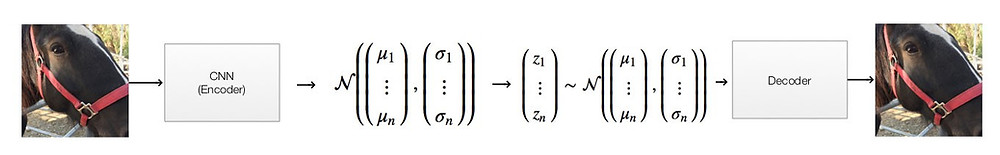### VERSA (paper)

To reduce the number of parameters to learn, the task context hᵢ generated is often used as the parameters of the last layer in a NN only, instead of generating parameters for the whole NN.Source (Terms)

In VERSA, we have a meta-learner model θ responsible for the feature extraction for the input x. Separately, it feeds the extracted features of the support to an amortization network (the light brown area below) to create a task context.Source

This learner approximates the posterior q over ψ, where ψ is the weights used in the last layer of a linear classifier.This learner is parameterized by 𝜙. To generate the weights, VERSA sample values from q, similar to the auto-encoder.

This layer multiplies the weights with the extracted features of the input. It generates a score which later normalized by the softmax for a probability distribution for our prediction y. It follows the concept in the Variational Auto-Encoder in defining and optimizing the loss function as:However, VERSA does not generate the weights for all classes at once. q𝜙(ψ | Dᵗ, θ) are trained in the context of a specific class, i.e. q𝜙(ψ | Dᵗ, θ, C) where C is the specific class. Therefore, q outputs the weight distribution for a single class (a single column in the matrix for the linear classifier) using the training examples for class C. It iterates the process for all classes to create the whole matrix in the linear classifier. (VERSA believes this weight generation will be more focused and easier.)### Amortized Bayesian Meta-Learning (paper)

The Bayesian concept can apply to the Meta-Learner Optimizer method also. Again, let us demonstrate it with an example with the graphical model defined on the right below.Source

The steps for solving this optimization problem are very similar to MAML. But the meta-leaner θ and learner 𝜙ᵢ (θᵢ’ above) will be modeled by probability distributions and the loss function will be calculated based on the variational inference.MAML: Source

In our example, let’s define the models for the prior, likelihood, and the variational posterior with probability distributions first. θ is composed of a mean and variance. Gaussian distribution and Gamma distribution are used to model the prior p(θ) for its mean and the variance respectively. And the likelihood p(𝜙ᵢ|θ) is modeled with a Gaussian centered around θ.The approximate posteriors q given the support of a task is modeled with a Gaussian.And the mean and the variance of 𝜙ᵢ approximated by q is computed by the SGD method.In this method, the learner is initialized with θ, i.e. we use the meta-learner as the starting point to refine the learner. Then, it is finetuned with k-step gradient descent to maximize the likelihood of the observed support (Dᵢ) for task i. SGD returns the variational parameters after K steps of gradient descent.The loss function we want to optimize is:where ψ is the variational parameters modeling p(θ). (But we will care less about ψ as we will drop it later).

Let’s simplified it a little bit more. In Meta-learning, the number of task M is huge but the number of samples N in each task is small (M ≫ N). So with far more tasks than the number of samples in each task, the uncertainty of θ is less significant and we can use a point estimate for θ instead. In terms of the probability distribution, this means:Where the probability equals 1 when θ equals θ* (θ* — MAP for θ). The KL terms KL(q(θ)||p(θ)) will be simplified as:And,

1. We don’t need to model the uncertainty of θ with ψ anymore and therefore, we can drop it.

2. In evaluation, we only use the support to estimate q. So for consistency between training and evaluation, the loss function can consider the support only.

With all these considerations, the loss function becomes: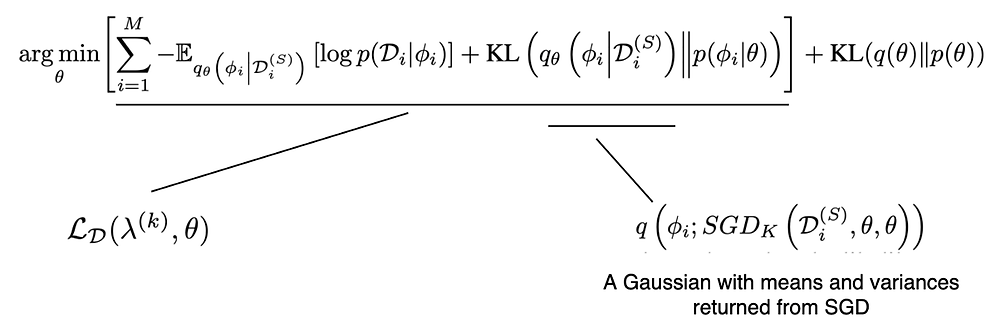For log p(Dᵢ | 𝜙), if 𝜙is a classifier, the term equals to the probability prediction for the true label. The qθ(𝜙ᵢ | Dᵢ)term is a Gaussian distribution with the mean and variance output by the SGD using k-step gradient descent. The first two terms below will be the loss function used in the SGD.

Now, we have what we need, and we can put them together as the algorithm below for the meta-training. In each episode (task), we compute the k-step SGD and it returns the mean and variance for 𝜙ᵢ (parameters modeling qθ(𝜙ᵢ | Dᵢ)). Afterward, we can use the loss function above to update θ.Modified from source

And, for reference, this is the algorithm for the meta-test.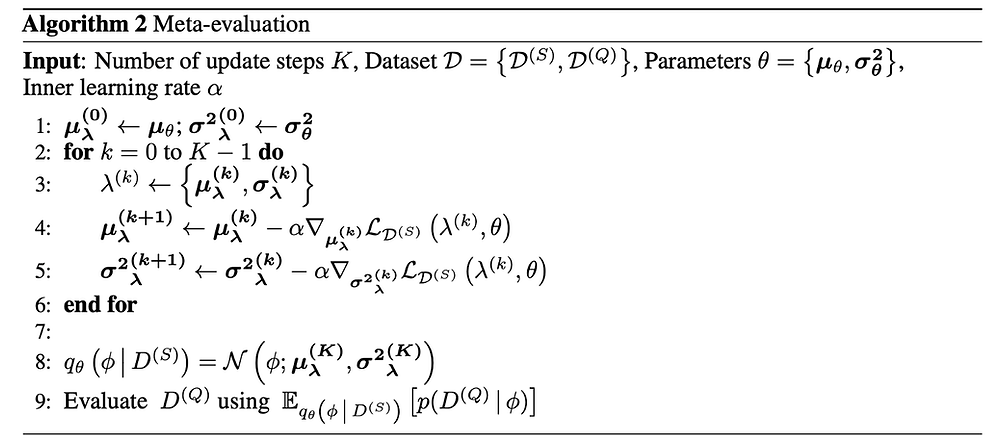### Stein Variational Gradient Descent (SVGD)

We will elaborate on a few more optimization-based methods with Bayesian. SVGD collects M samples from distribution p(θ). These M samples serve as M instances of models that each can make predictions. Such an instance is called a particle. The general idea is to allow these particles to evolve using gradient descents. In the end, we use the average of their predictions as output. We can think of this as an ensemble method with different trained models.

At iteration t, each particle is updated by the following rule:Each particle consults with other particles on their gradients to determine its own update direction with weights computed from a kernel (a.k.a. measuring the similarity), with larger influences from the nearby particles. The last term above acts as a repulsive force that avoids particles to collapse to a single point. We just want to demonstrate the core concept and please refer to the original paper to understand the equation.

To make predictions, we sample from these particles and make predictions from these models. The output will be the average of these predictions.### Bayesian Meta-Learning with Chaser Loss (BMAML)

BMAML is built with multiple steps SVGD above with the following algorithm.Its objective can be viewed as making the task-train posterior to be as close to the true task-train posterior that runs infinite SVGD steps. Since we cannot perform infinite steps, we will approximate it by taken s steps. So at least, it gives us some directions.Therefore, to move towards the true task-train posterior, BMAML performs an addition s steps of SVGD. The cost function will measure the dissimilarity between n steps and n+s steps:In short, BMAML computes the gradient of the dissimilarity of the chaser and the leader and updates the model parameter Θ with gradient descent.

### Probabilistic Model-Agnostic Meta-Learning (paper)

For the last probabilistic model, we will introduce stochastic to traditional MAML — the Probabilistic MAML. Let’s start with a graphical model below that demonstrates the meta-learning dependency.In MAML, θ acts as the meta-learner model parameters, say using a NN, and 𝜙ᵢ is the adapted model specialized for task i. In many meta-learning algorithms, we only model the last layer in NN in adapting to new tasks. The limitation is mainly caused by small sample size and computation complexity. In this section, we will incorporate stochastic to model uncertainty better, especially needed for the small sample size. On top, the method will have lower complexity and therefore we may be able to model the whole model.

As shown below, the posterior inference over 𝜙ᵢ will depend on p(θ), p(𝜙ᵢ | θ), and the support. Unfortunately, it is in general intractable.But if we can estimate or infer p(𝜙ᵢ | θ, D_train) directly, the solution will be much easier. Indeed, we have done it a few times of optimizing 𝜙ᵢ using gradient descent in the inner loop (the SGD below).Once p(𝜙ᵢ | θ, D_train) can be inferred (approximated), the graphical model will be transformed into the one on the right. One important observation is that θ does not depend on x_train and y_train anymore — we will use this to simplify the equations later.Modified from source

Let’s jump to the Probabilistic MAML algorithm to see what we need. The red lines are the code added for the Probabilistic MAML.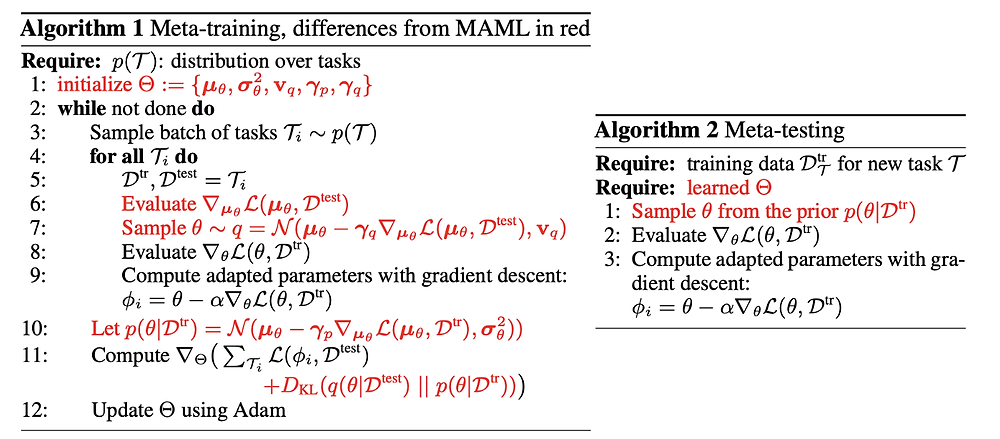One major difference is the model θ that we start with for the task adoption (lines 6 and 7). The second is the remodeling of p(θ | D_training) (line 10) and how we compute the loss with the variational inference (line 11).

First, we will model p(θ) as a Gaussian with a learned mean and diagonal covariance. We can also model p(𝜙ᵢ|θ) with a Gaussian.Before the inner loop gradient descent, MAML initializes 𝜙ᵢ with θ (a point estimate). Now, let makes it even better. We can readjust θ according to the evidence before passing it to 𝜙ᵢ in the original MAML algorithm.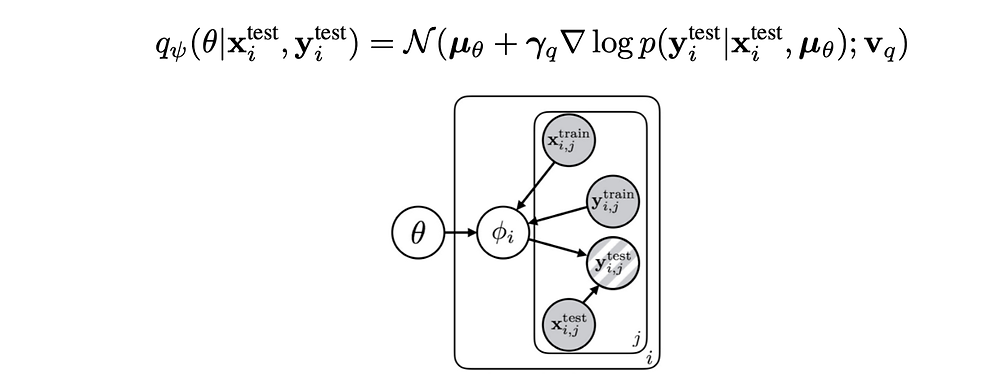Source

As discussed before, with the transformed graphical model above, θ does not depend on the training data. Therefore the posterior (θ | evidence) can be simplified as (θ | x_test, y_test). One possible estimation for the posterior q is to reuse the learned mean of the prior µθ and readjust it with the gradient loss.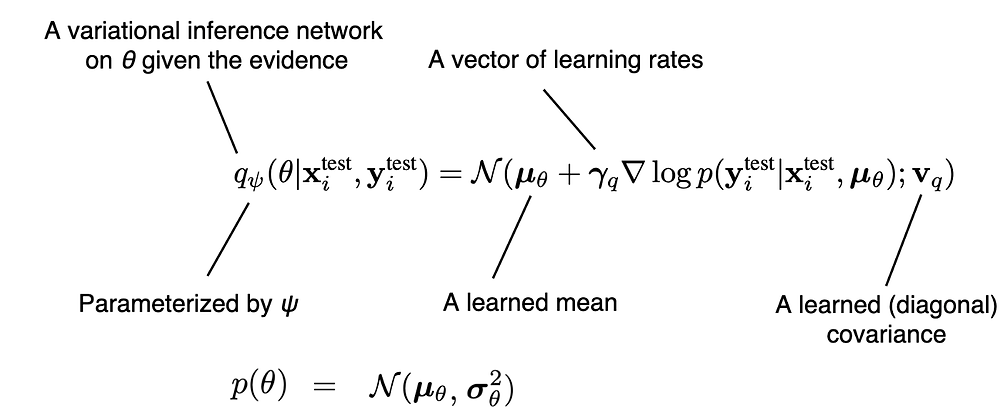So instead of using θ as the initial model to be adapted, we sample a model θ with q and use that for the initial model to be adapted.Next, we will define the training loss function. MAML can be interpreted as approximate inference for the posterior below:Where 𝜙ᵢ* is the MAP.If the likelihood is Gaussian in 𝜙ᵢ, gradient descent for a fixed number of iterations corresponds to MAP inference under a Gaussian prior p(𝜙ᵢ|θ). In short, the MAP 𝜙ᵢ* in the equation above can be estimated by the Gradient Descent, the same in the MAML’s inner loop (the adapted model 𝜙ᵢ).Conventional MAML can be viewed as approximating p(𝜙ᵢ | θ, evidence) as a Dirac function δ with 𝜙ᵢ*.In general, this is a crude approximation but once we can infer p(𝜙ᵢ | θ, evidence), the loss function becomes more tractable. Recall the general lower bound estimation (the loss function) for the variation inference is formulated as: